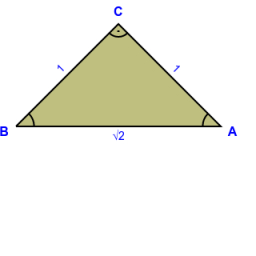# Calculate 68454

Calculate how many times the square of the number 1/100 is less than 1/1000. (numbers in fraction form)

n =  10

### Step-by-step explanation:Did you find an error or inaccuracy? Feel free to write us. Thank you!

Tips for related online calculators
Need help calculating sum, simplifying, or multiplying fractions? Try our fraction calculator.